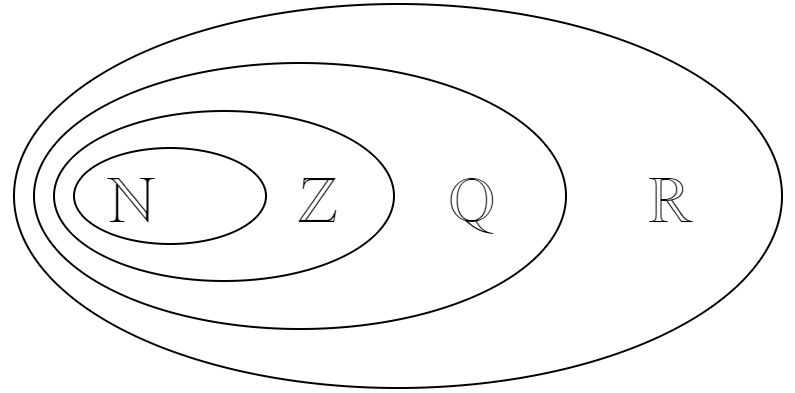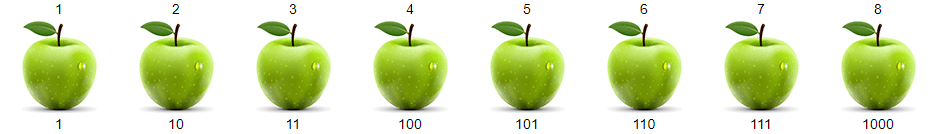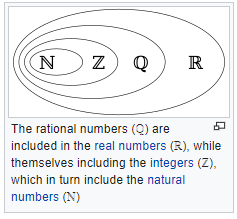Closed Loop Interval OntologyCLOSED LOOP INTERVAL ONTOLOGY        The Digital Integration of Conceptual FormTzimTzum/Kaballah | Loop definition | Home | ORIGINPlease sign in or register Email * Password * Searches selected display Universal conceptual form Aligning the vision Evolving and coalescing Why we do this Ethics / governance / science Homeostatic governance Idealized democracy Reconciliation and integration Holistic view on alternatives Definitions and alternatives Compare alternatives How do we know? How meaning is created Foundational recursive definition Spectrum of levels The closed loop ensemble contains all primary definitions Dimensions of set theory What is a number? Topology of sets How are they constructed? Primary terms Closed Loop framework Hierarchical models Euclid in digital space Foundational method Compositional semantics How it works The integrated science of mind What does it mean? Core terms Constructive elements and building blocks Preserving data under transformation In the beginning Domain and universal A design proposal Mapping the grid From other sources Foundational computation Perfecting political balance Simultaneity or sequence Concrete symbolic representation Science, philosophy and math are defined in concepts Emerging from a pointNumbers
What is a number?

 We want to construct our definition of number in terms that are consistent with synthetic dimensionality and the constraint on the term "real" by requiring "actuality" N Natural number Z Integer Q Rational R Real Let's look at "natural number" -- which is illustrated in Wikipedia by apples. Apples are good objects to illustrate this argument, because we could also illustrate numbers with oranges, or some other kind of fruit. We want to look into this -- following Tobias Dantzig -- and probably a few others, including Queen and Servant of Science Let's look into the thesis that perhaps "no number is real that is not actual" Actual numbers are numbers that can measure and describe "actual things" [Is this an adequate definition? Tobias Dantzig says that working with imaginary numbers "produce concrete results" (intro, p.ix)] Under this view, there are no actual "negative numbers". Negative numbers are computational artefacts. The actual numbers are "natural numbers" and "integers" and fractionsNatural number Natural number -- basis for countingMon, May 10, 2021 Reference In mathematics, the natural numbers are those used for counting (as in "there are six coins on the table") and ordering (as in "this is the third largest city in the country"). In common mathematical terminology, words colloquially used for counting are "cardinal numbers", and words used for ordering are "ordinal numbers". The natural numbers can, at times, appear as a convenient set of codes (labels or "names"), that is, as what linguists call nominal numbers, forgoing many or all of the properties of being a number in a mathematical sense. The set of natural numbers is often denoted by the symbol N **** Two important generalizations of natural numbers arise from the two uses of counting and ordering: cardinal numbers and ordinal numbers. A natural number can be used to express the size of a finite set; more precisely, a cardinal number is a measure for the size of a set, which is even suitable for infinite sets. This concept of "size" relies on maps between sets, such that two sets have the same size, exactly if there exists a bijection between them. The set of natural numbers itself, and any bijective image of it, is said to be countably infinite and to have cardinality aleph-null (?0). Natural numbers are also used as linguistic ordinal numbers: "first", "second", "third", and so forth. This way they can be assigned to the elements of a totally ordered finite set, and also to the elements of any well-ordered countably infinite set. This assignment can be generalized to general well-orderings with a cardinality beyond countability, to yield the ordinal numbers. An ordinal number may also be used to describe the notion of "size" for a well-ordered set, in a sense different from cardinality: if there is an order isomorphism (more than a bijection!) between two well-ordered sets, they have the same ordinal number. The first ordinal number that is not a natural number is expressed as ?; this is also the ordinal number of the set of natural numbers itself. *********** In mathematics, the natural numbers are those used for counting (as in "there are six coins on the table") and ordering (as in "this is the third largest city in the country"). In common mathematical terminology, words colloquially used for counting are "cardinal numbers", and words used for ordering are "ordinal numbers". The natural numbers can, at times, appear as a convenient set of codes (labels or "names"), that is, as what linguists call nominal numbers, forgoing many or all of the properties of being a number in a mathematical sense. The set of natural numbers is often denoted by the symbol {\displaystyle \mathbb {N} }\mathbb {N} . Some definitions, including the standard ISO 80000-2,[a] begin the natural numbers with 0, corresponding to the non-negative integers 0, 1, 2, 3, ... (sometimes collectively denoted by the symbol {\displaystyle \mathbb {N} _{0}}\mathbb {N} _{0}, to emphasize that zero is included), whereas others start with 1, corresponding to the positive integers 1, 2, 3, ... (sometimes collectively denoted by the symbol {\displaystyle \mathbb {N} _{1}}\mathbb {N} _{1}, {\displaystyle \mathbb {N} ^{+}}{\displaystyle \mathbb {N} ^{+}}, or {\displaystyle \mathbb {N} ^{*}}{\mathbb {N}}^{*} for emphasizing that zero is excluded).[b] Texts that exclude zero from the natural numbers sometimes refer to the natural numbers together with zero as the whole numbers, while in other writings, that term is used instead for the integers (including negative integers).[dubious – discuss] The natural numbers are a basis from which many other number sets may be built by extension: the integers, by including (if not yet in) the neutral element 0 and an additive inverse (?n) for each nonzero natural number n; the rational numbers, by including a multiplicative inverse ( 1 / n ) for each nonzero integer n (and also the product of these inverses by integers); the real numbers by including with the rationals the limits of (converging) Cauchy sequences of rationals; the complex numbers, by including with the real numbers the unresolved square root of minus one (and also the sums and products thereof); and so on.[c][d] These chains of extensions make the natural numbers canonically embedded (identified) in the other number systems. Properties of the natural numbers, such as divisibility and the distribution of prime numbers, are studied in number theory. Problems concerning counting and ordering, such as partitioning and enumerations, are studied in combinatorics. In common language, particularly in primary school education, natural numbers may be called counting numbers to intuitively exclude the negative integers and zero, and also to contrast the discreteness of counting to the continuity of measurement — a hallmark characteristic of real numbers.

 Integer number Emerging/exploratory claim -- is that "there are no negative integers" or "negative numbers" negative numbers are computational abstractions and artefacts of computation as compuational objects, yes they exist, but they are not "real" in the sense of "actual" Sun, May 2, 2021 Reference An integer (from the Latin integer meaning "whole")[a] is colloquially defined as a number that can be written without a fractional component. For example, 21, 4, 0, and ?2048 are integers, while 9.75, 5+ 1 / 2 , and ?2 are not. The set of integers consists of zero (0), the positive natural numbers (1, 2, 3, ...), also called whole numbers or counting numbers, and their additive inverses (the negative integers, i.e., ?1, ?2, ?3, ...). The set of integers is often denoted by the boldface (Z) or blackboard bold {\displaystyle (\mathbb {Z} )}{\displaystyle (\mathbb {Z} )} letter "Z"—standing originally for the German word Zahlen ("numbers"). ? is a subset of the set of all rational numbers ?, which in turn is a subset of the real numbers ?. Like the natural numbers, ? is countably infinite. The integers form the smallest group and the smallest ring containing the natural numbers. In algebraic number theory, the integers are sometimes qualified as rational integers to distinguish them from the more general algebraic integers. In fact, (rational) integers are algebraic integers that are also rational numbers.

 Ordinal number Ordinal number Sun, May 2, 2021 Reference https://en.wikipedia.org/wiki/Ordinal_number In set theory, an ordinal number, or ordinal, is one generalization of the concept of a natural number that is used to describe a way to arrange a (possibly infinite) collection of objects in order, one after another. Any finite collection of objects can be put in order just by the process of counting: labeling the objects with distinct natural numbers. The basic idea of ordinal numbers is to generalize this process to possibly infinite collections and to provide a "label" for each step in the process. Ordinal numbers are thus the "labels" needed to arrange collections of objects in order. An ordinal number is used to describe the order type of a well-ordered set (though this does not work for a well-ordered proper class). A well-ordered set is a set with a relation < such that: (Trichotomy) For any elements x and y, exactly one of these statements is true: x < y y < x x = y (Transitivity) For any elements x, y, z, if x < y and y < z, then x < z. (Well-foundedness) Every nonempty subset has a least element, that is, it has an element x such that there is no other element y in the subset where y < x.

 The basket/container model of an integer/natural number We want to show in simple terms how the number 3 can be understood as something like a basket or a container for three identical units -- like "three apples" Sun, May 2, 2021

 Cardinal number Number for measuring the size of a set -- "the number of objects in the set" Sun, May 2, 2021 Reference In mathematics, cardinal numbers, or cardinals for short, are a generalization of the natural numbers used to measure the cardinality (size) of sets. The cardinality of a finite set is a natural number: the number of elements in the set. The transfinite cardinal numbers, often denoted using the Hebrew symbol {\displaystyle \aleph }\aleph (aleph) followed by a subscript, describe the sizes of infinite sets. Cardinality is defined in terms of bijective functions. Two sets have the same cardinality if, and only if, there is a one-to-one correspondence (bijection) between the elements of the two sets. In the case of finite sets, this agrees with the intuitive notion of size. In the case of infinite sets, the behavior is more complex. A fundamental theorem due to Georg Cantor shows that it is possible for infinite sets to have different cardinalities, and in particular the cardinality of the set of real numbers is greater than the cardinality of the set of natural numbers. It is also possible for a proper subset of an infinite set to have the same cardinality as the original set—something that cannot happen with proper subsets of finite sets. There is a transfinite sequence of cardinal numbers: {\displaystyle 0,1,2,3,\ldots ,n,\ldots ;\aleph _{0},\aleph _{1},\aleph _{2},\ldots ,\aleph _{\alpha },\ldots .\ }0,1,2,3,\ldots ,n,\ldots ;\aleph _{0},\aleph _{1},\aleph _{2},\ldots ,\aleph _{\alpha },\ldots .\ This sequence starts with the natural numbers including zero (finite cardinals), which are followed by the aleph numbers (infinite cardinals of well-ordered sets). The aleph numbers are indexed by ordinal numbers. Under the assumption of the axiom of choice, this transfinite sequence includes every cardinal number. If one rejects that axiom, the situation is more complicated, with additional infinite cardinals that are not alephs. Cardinality is studied for its own sake as part of set theory. It is also a tool used in branches of mathematics including model theory, combinatorics, abstract algebra and mathematical analysis. In category theory, the cardinal numbers form a skeleton of the category of sets.

 Whole numberMon, May 10, 2021

 Rational numberEdited from sec=201 ?sec=201&tm=100950#ctx 5/10/2021 In mathematics, a rational number is a number such as 3/7 that can be expressed as the quotient or fraction p/q of two integer Mon, May 10, 2021 Reference In mathematics, a rational number is a number such as 3/7 that can be expressed as the quotient or fraction p/q of two integers, a numerator p and a non-zero denominator q. Every integer is a rational number: for example, 5 = 5/1. The set of all rational numbers, also referred to as "the rationals", the field of rationals or the field of rational numbers is usually denoted by a boldface Q (or blackboard bold {\displaystyle \mathbb {Q} }\mathbb {Q} , Unicode ??/?); it was thus denoted in 1895 by Giuseppe Peano after quoziente, Italian for "quotient".

 Real number Mon, May 10, 2021

 Arithmetic Addition, subtraction, multiplication, division Hierarchy of number types -- from simple to complex How numbers are defined by synthetic dimensions Keep this very brief, and get into more detail in the Arithmetic Theme Group Thu, May 6, 2021 Reference Arithmetic (from the Greek arithmos, 'number' and, tiké [téchne], 'art' or 'craft') is a branch of mathematics that consists of the study of numbers, especially the properties of the traditional operations on them—addition, subtraction, multiplication, division, exponentiation and extraction of roots. Arithmetic is an elementary part of number theory, and number theory is considered to be one of the top-level divisions of modern mathematics, along with algebra, geometry, and analysis. The terms arithmetic and higher arithmetic were used until the beginning of the 20th century as synonyms for number theory, and are sometimes still used to refer to a wider part of number theory.

 Indexing levels of distinctions define types of numbers in classes along the y axis, the numbers themselves as an extension in the x axis 1) the continuum 2) binary numbers -- the first slice into the continuum (note -- binary numbers are shown in a single line or dimension -- but they are actually opposites 3) whole numbers (decimal system or whatever) 4) decimal numbers 5) boolean objects -- composite constructions (?) 6) alphabets 7) wordsWed, May 12, 2021